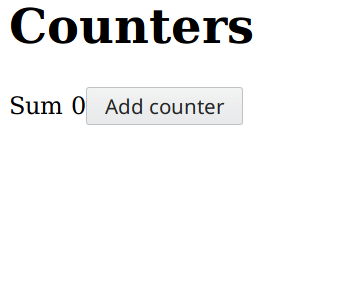Geek Repo

Github PK Tool

# purescript-turbine

• Based on higher-order FRP with support for continuous time.
• Concise and powerful thanks to FRP.
• No big global state/model. Everything is encapsulated in components.
• Type-safe communication between views and models.
• Model logic and view code is kept separate for logic-less views.
• Highly modular and composable thanks to encapsulated stateful components.
• Avoids using virtual DOM by utilizing FRP to make all changes to the DOM in reaction to changing behaviors.
• Easy to reason about thanks to tractable reactive data flow.

## Inline Examples

### Single counter```counter id = component \on -> do
count <- accum (+) 0 on.change
( H.div {} (
H.text "Counter " </>
H.span {} (H.textB \$ map show count) </>
H.button {} (H.text "+" ) `use` (\o -> { change: o.click \$> 1 }) </>
H.button {} (H.text "-" ) `use` (\o -> { change: o.click \$> -1 })
)
) `output` {}

main = runComponent "#mount" (counter 0)```

### List of counters

Show a list of counters. New counters can be added to the list. Existing counters can be deleted. The aggregated sum of all the counters is shown.```counter id = component \on -> do
count <- accum (+) 0 on.change
( H.div {} (
H.text "Counter " </>
H.span {} (H.textB \$ map show count) </>
H.button {} (H.text "+" ) `use` (\o -> { change: o.click \$> 1 }) </>
H.button {} (H.text "-" ) `use` (\o -> { change: o.click \$> -1 }) </>
H.button {} (H.text "x") `use` (\o -> { delete: o.click })
)
) `output` { count, delete: on.delete \$> id }

counterList init = component \on -> do
let sum = on.listOut >>= (map (_.count) >>> foldr (lift2 (+)) (pure 0))
let removeId = map (fold <<< map (_.delete)) on.listOut
let removeCounter = map (\i -> filter (i /= _)) (shiftCurrent removeId)
nextId <- scan (+) 0 (on.addCounter \$> 1)
let appendCounter = cons <\$> nextId
counterIds <- accum (\$) init (appendCounter <> removeCounter)
( H.div {} (
H.h1 {} (H.text "Counters") </>
H.span {} (H.textB (map (\n -> "Sum " <> show n) sum)) </>
H.button {} (H.text "Add counter") `use` (\o -> { addCounter: o.click }) </>
list (\id -> counter id `use` identity) counterIds identity `use` (\o -> { listOut: o })
)
) `output` {}

main = runComponent "#mount" (counterList )```

## Installation

The following installs Hareactive and Turbine. Hareactive is the FRP library that Turbine builds upon and is a hard dependency.

``````npm i @funkia/hareactive
bower install --save purescript-hareactive
npm i @funkia/turbine
bower install --save purescript-turbine
``````

Alternatively, use the purescript-turbine-starter, a project template that contains Turbine and Hareactive pre-setup.

## Documentation

The best place to start is the getting started tutorial. It is a quick start tutorial which aims to explain the basics of Turbine as briefly as possible.

There is also an older, slightly outdated, tutorial.

Both API documentation for Turbine and API documentation for Hareactive is on Pursuit.

Taking a look at the examples is also a great way to see Turbine used in practice and to see how specific things are accomplished.

## Examples

• Email validator -- A simple email validator.
• Fahrenheit celsius converter -- Conversion between fahrenheit and celsius.
• Counters -- A list of counters. This example shows how to create a dynamic list of components.
• Continuous time -- A very simple example showing how to work with continuous time.
• Timer -- A more complicated example demonstrating continuous time by implementing a timer with a progress bar.
• Zip codes -- A zip code validator. Shows how to perform `Effect`s using FRP.
• TodoMVC -- The classic TodoMVC example (still has a couple of bugs and no routing).